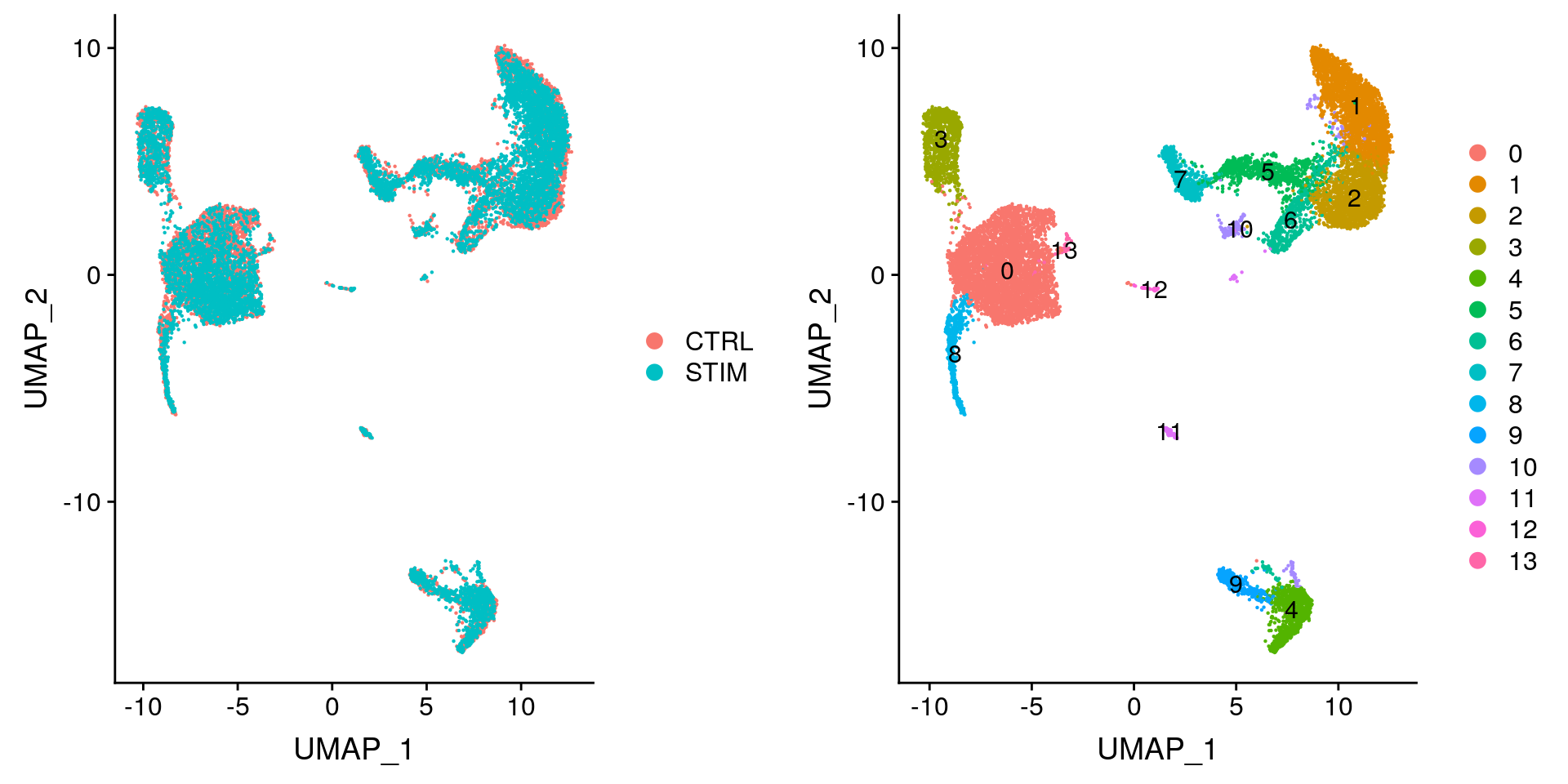This tutorial walks through an alignment of two groups of PBMCs from Kang et al, 2017. In this experiment, PBMCs were split into a stimulated and control group and the stimulated group was treated with interferon beta. The response to interferon caused cell type specific gene expression changes that makes a joint analysis of all the data difficult, with cells clustering both by stimulation condition and by cell type. Here, we demonstrate our integration strategy, as described in Stuart and Butler et al, 2018, for performing integrated analyses to promote the identification of common cell types and enable comparative analyses. While this example demonstrates the integration of two datasets (conditions), these methods have been extended to multiple datasets. This workflow provides an example of integrating four pancreatic islet datasets.

### Integration goals

The following tutorial is designed to give you an overview of the kinds of comparative analyses on complex cell types that are possible using the Seurat integration procedure. Here, we address three main goals:

• Identify cell types that are present in both datasets
• Obtain cell type markers that are conserved in both control and stimulated cells
• Compare the datasets to find cell-type specific responses to stimulation

### Setup the Seurat objects

The gene expression matrices can be found here. We first read in the two count matrices and set up the Seurat objects.

``````library(Seurat)
library(cowplot)
ctrl.data <- read.table(file = "../data/immune_control_expression_matrix.txt.gz", sep = "\t")
stim.data <- read.table(file = "../data/immune_stimulated_expression_matrix.txt.gz", sep = "\t")

# Set up control object
ctrl <- CreateSeuratObject(counts = ctrl.data, project = "IMMUNE_CTRL", min.cells = 5)
ctrl\$stim <- "CTRL"
ctrl <- subset(ctrl, subset = nFeature_RNA > 500)
ctrl <- NormalizeData(ctrl, verbose = FALSE)
ctrl <- FindVariableFeatures(ctrl, selection.method = "vst", nfeatures = 2000)

# Set up stimulated object
stim <- CreateSeuratObject(counts = stim.data, project = "IMMUNE_STIM", min.cells = 5)
stim\$stim <- "STIM"
stim <- subset(stim, subset = nFeature_RNA > 500)
stim <- NormalizeData(stim, verbose = FALSE)
stim <- FindVariableFeatures(stim, selection.method = "vst", nfeatures = 2000)``````

### Perform integration

We then identify anchors using the `FindIntegrationAnchors` function, which takes a list of Seurat objects as input, and use these anchors to integrate the two datasets together with `IntegrateData`.

``immune.anchors <- FindIntegrationAnchors(object.list = list(ctrl, stim), dims = 1:20)``
``immune.combined <- IntegrateData(anchorset = immune.anchors, dims = 1:20)``

### Perform an integrated analysis

Now we can run a single integrated analysis on all cells!

``````DefaultAssay(immune.combined) <- "integrated"

# Run the standard workflow for visualization and clustering
immune.combined <- ScaleData(immune.combined, verbose = FALSE)
immune.combined <- RunPCA(immune.combined, npcs = 30, verbose = FALSE)
# t-SNE and Clustering
immune.combined <- RunUMAP(immune.combined, reduction = "pca", dims = 1:20)
immune.combined <- FindNeighbors(immune.combined, reduction = "pca", dims = 1:20)
immune.combined <- FindClusters(immune.combined, resolution = 0.5)``````
``````# Visualization
p1 <- DimPlot(immune.combined, reduction = "umap", group.by = "stim")
p2 <- DimPlot(immune.combined, reduction = "umap", label = TRUE)
plot_grid(p1, p2)``````To visualize the two conditions side-by-side, we can use the `split.by` argument to show each condition colored by cluster.

``DimPlot(immune.combined, reduction = "umap", split.by = "stim")``